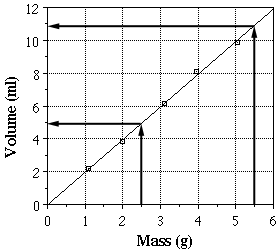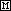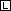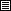Interpolation and Extrapolation

## Interpolation and Extrapolation

Besides being able to show trends between variables, plotting data on a graph allows us to predict values for which we have taken no data. When we predict values that fall within the range of data points taken it is called interpolation. When we predict values for points outside the range of data taken it is called extrapolation. Extrapolation over too far a range can be dangerous unless it is certain that the relationship between the variables continues over the entire range.

Consider these examples based on the volume/mass data from the previous page. We could use our graph to interpolate the volume for a sample with a mass of 2.5 g. This is done by drawing a vertical line from the x-axis at a value of 2.5 g until it crosses our best fit line, and then drawing a horizontal line to the y-axis. The y-value at this point, 4.9 ml, is equal to the volume of 2.5 g sample. The same process is used for extrapolation. A sample with a mass of 5.5 g, will have a volume of 10.8 ml.These values could also be determined using the equation for the best fit line determined previously.

volume = 1.982 (mass) + 0.002

If 2.5 g is substituted for the mass, the calculated volume will be 4.96 ml. If a mass of 5.5 g is used, the volume will be 10.90 ml. Both of these values are close to those read off the graph.

Note that while in both of the above examples we determined the volume from a given mass, the opposite could also be performed. If a sample has a volume of 7.0 ml, we could determine that its mass should be 3.53 g.